## 10 Apr 2016

### Agler (4.1) Symbolic Logic: Syntax, Semantics, and Proof, "Truth-Tree Setup and Basics in Decomposition", summary

by Corry Shores
[Search Blog Here. Index-tags are found on the bottom of the left column.]

[The following is summary. Boldface (except for metavariables) and bracketed commentary are my own. Please forgive my typos, as proofreading is incomplete. I highly recommend Agler’s excellent book. It is one of the best introductions to logic I have come across.]

Summary of

David W. Agler

Symbolic Logic: Syntax, Semantics, and Proof

Ch.4: Truth Trees

4.1 Truth-Tree Setup and Basics in Decomposition

Brief Summary:
We will conduct decision procedures using truth-trees. There are three steps in this method: (a) set up the tree for decomposition, (b) decompose decomposable propositions into a non-decomposable form using the decomposition rules, and (c) analyze the completed truth-tree for certain logical properties. The trees are structured with three columns. The left one enumerates the line. The center one gives the proposition. And the right one lists the decomposition rule along with the line where the proposition we are decomposing was previously located. There are nine types of propositions that we can decompose: conjunction, disjunction, conditional, biconditional, negated conjunction [¬(P∧R)], negated disjunction [¬(P∨R)], negated conditional [¬(P→R)], negated biconditional [¬(P↔R)], and double negation. There are three sorts of decompositional patterns: (a) stacking, for when the proposition is true under just one truth-value assignment, (b) branching, for when the proposition is false under just one truth-value assignment, or (c) stacking and branching, for when the proposition is true under two truth-value assignments and false under two truth-value assignments.

Summary

Ch.4: Truth Trees

In the previous chapter, we used decision procedures implementing truth-tables to test propositions and arguments for certain logical properties, namely, tautology, contradiction, contingency, consistency, equivalence, and validity. One problem with the truth-table methodology is that it can become unmanageable when we have more than three propositional terms (99). So we now will examine decision procedures that use truth- trees rather than truth-tables, because here the decision procedures can be carried out more efficiently.

Also, in a later chapter when we deal with predicate logic, the truth-table method will not be suitable, “because predicate logic is not a truth-functional language. However, it will be possible to make use of the truth-tree method as a partial decision procedure” (99).

4.1 Truth-Tree Setup and Basics in Decomposition

There are three steps in the truth-tree method:

(1) Set up the truth tree for decomposition.
(2) Decompose any propositions into a nondecomposable form using decomposition rules.
(3) Analyze the completed truth tree for a specified logical property.
(quoting Agler 99)

First we will need to discuss some terminology. Second we will learn how we construct truth trees. And third we will see how to analyze the trees for certain logical properties (100).

4.1.1 Truth-Tree Setup

Agler’s truth-trees are structured with three columns. The first one merely gives the number for the propositions that are provided in the second column. Thus the second column is where we find the propositions that we are decomposing. And the third column tells us which proposition (from a previous line) is being decomposed along with the justification for that decomposition (100).

[Recall step 1 from above was “Set up the truth tree for decomposition”.] So in the first step, we will vertically stack the and number the propositions. We consider as an illustration these two propositions:

(R∧¬M), R∧(W∧¬M)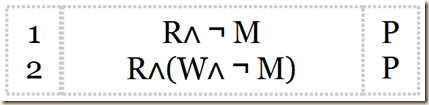It does not matter what order we stack them. The justification letter “P” means “proposition” “to indicate that this particular proposition is part of the set of propositions provided” (100).

4.1.2 Truth-Tree Decomposition

[Recall that step 2 is: “Decompose any propositions into a nondecomposable form using decomposition rules”.] Now we move to step 2 where we decompose the propositions that are in a decomposable form. There are nine types of propositions that have such a form allowing for their decomposition:

There are three sorts of decompositional patterns: (a) stacking, (b) branching, or (c) stacking and branching. These decompositions are conducted in accordance with the decomposition rules. Agler will first given an abstract account of how the rules are formatted.

We will use a generic truth-functional operator sign #, and we will consider P#Q to be a decomposable proposition. A stacking decomposition looks like this: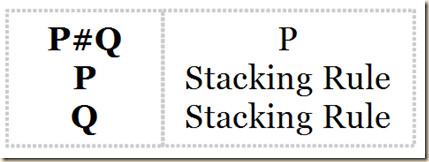A branching decomposition looks like: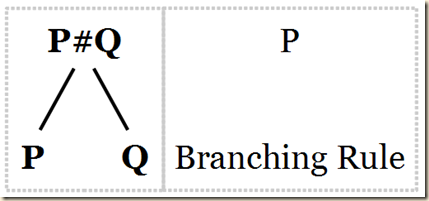And a branching and stacking decomposition will look like: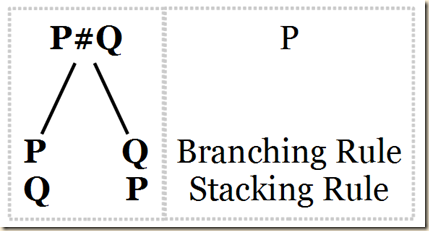Agler explains that what determines whether we stack, branch, or branch and stack when decomposing depends on the truth conditions for the proposition. We stack whenever the proposition is true under just one truth-value assignment.

Stacking rule: A stacking rule is a truth-tree rule where the condition under which a proposition ‘P’ is true is represented by stacking. A stacking rule is applied to propositions that are true under one truth-value assignment.
(101)

Agler then presents this table so he can explain the matter more clearly.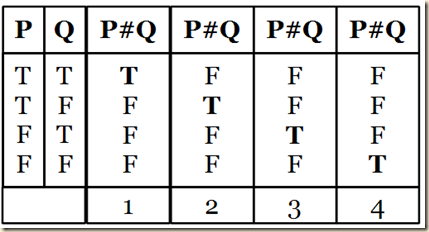As we can see, each P#Q is true only under one truth-value assignment. As we noted, this means that the each would be decomposed using a stacking rule.

So consider the first column. Here the whole proposition is evaluated as true if both terms are assigned the value of true. That means we would stack P above Q (102). We can think of it as meaning: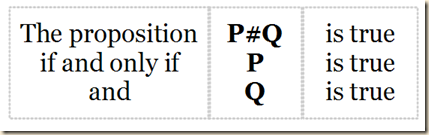Now instead consider column 2. Here the proposition is evaluated as true if P is assigned the value of true and Q is assigned the value of false. In this case, we would stack P above ¬Q (102). Here we can think of it as meaning: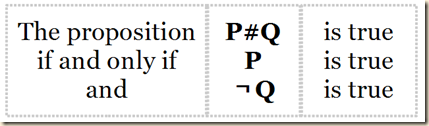Branching decompositions happen when the proposition is false under just one truth-value assignment.

Branching rule: A branching rule is a truth-tree rule where the condition under which a proposition ‘P’ is true is represented by branching. A branching rule is applied to propositions that are false only under one truth-value assignment.
(103)

So consider this table: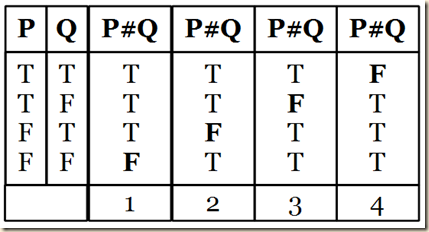See how in column 1, the whole proposition is false only when both terms are false. And it is true so long as at least one of the two terms are true. This means we will decompose it with P at the end of one branch and Q at the end of the other (103). We can think of it as saying: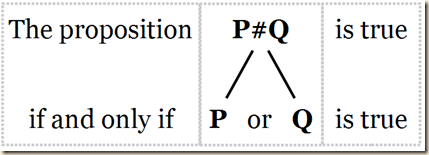And like before, if one of the terms is false in the assignment, it would be negated on the branching.

We use branching and stacking decomposition “when the proposition is true under two truth- value assignments and false under two truth-value assignments” (104).

Branching-and-stacking rule: A branching-and-stacking rule is a truth-tree rule where the truth conditions of a proposition ‘P’ are represented by branching and stacking. A branching- and-stacking rule will be used when ‘P’ is true under only two truth-value  assignments and false under two truth-value assignments.
(104)

Here is the table for this pattern: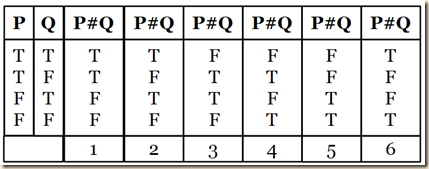Agler then says that “The representation of these truth functions in terms of a truth tree is left as an exercise” (104). [The problem is that I am not sure how to do this. I will propose a possibility, and I will explain why I am not sure about it. Let us do column 6, because that would be the biconditional operator. One representation that I think could possibly work is: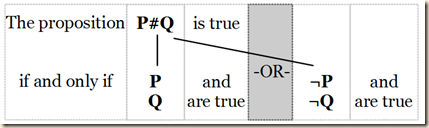But this is not structured like the other ones. We should have a simpler structure that follows the other patterns. However, I do not think it works if we do this: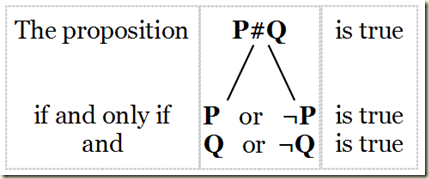I am pretty sure that the biconditional P↔Q does not mean ((P∨¬P)∧(Q∨¬Q)), because they are not logically equivalent, and the second one is a tautology anyway. However, I am assuming that we should have something in this simpler form. I am just not sure how to do it.]

Agler, David. Symbolic Logic: Syntax, Semantics, and Proof. New York: Rowman & Littlefield, 2013.

.